# Cervical-Occipital Assembly Movements of the Upper Cervical Assembly

 Page 3/14 Date 23.04.2018 Size 1.62 Mb. #45829

## Quaternions

It can be shown that if the axis of a rotation,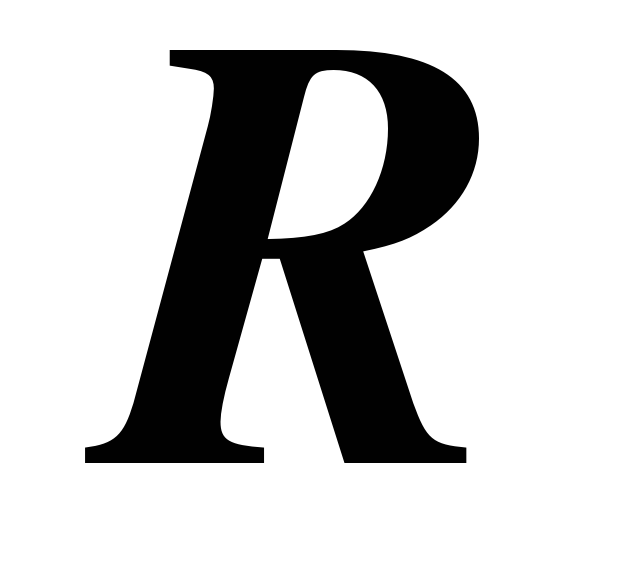, is aligned with the vector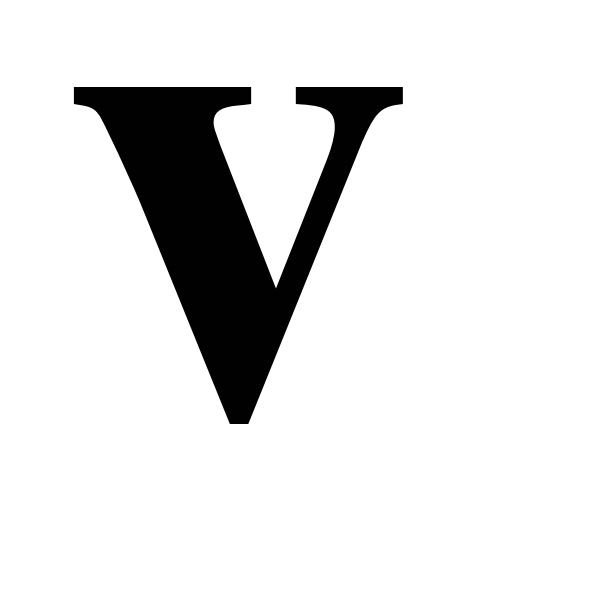and the rotation is through an angle, then a structure aligned with the vectorwill be changed into the vectorby the rotation, whereis given by the following expression.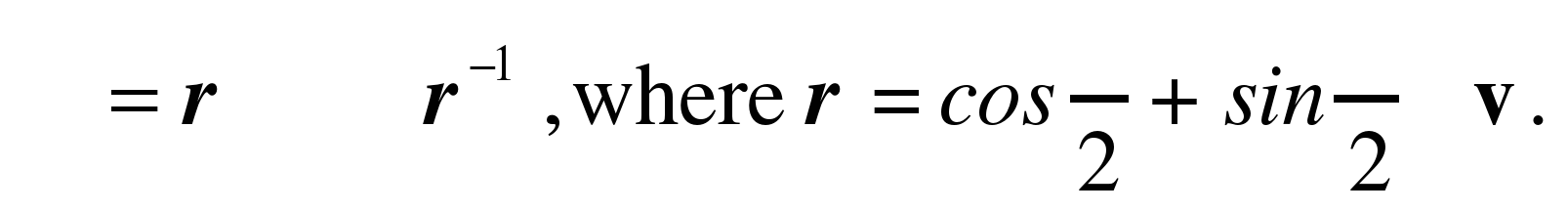The variable r is a quaternion, which is a hypercomplex number that is the sum of a scalar and a vector. Quaternions behave much like other algebraic numbers except that the vector is expressed as a sum of three different imaginary numbers, corresponding to the three coordinate axes. The basis vectors are i, j, and k. A vector is expressed as a sum of real multiples of these basis vectors, so a vector that extends two units anterior, three units lateral, and five units superior would be written as: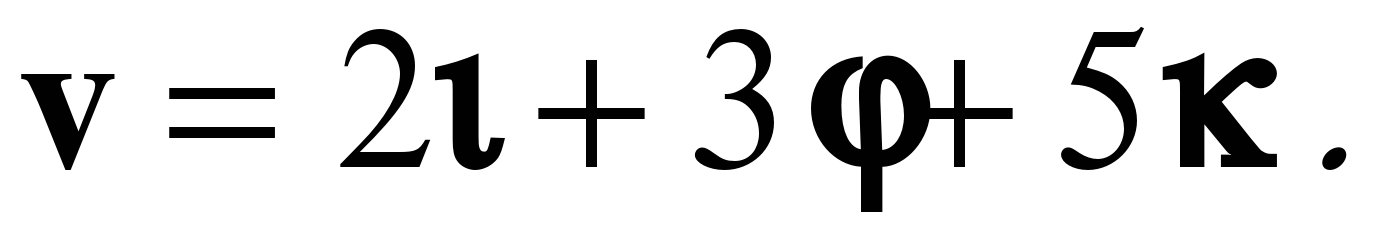The unusual feature of quaternions is that since i, j, and k are three different imaginary numbers their products are as follows.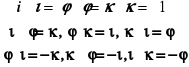The bases are imaginary numbers because their squares are equal to –1; they are different because the product of any two is the third, and the order of multiplication is relevant. As strange as this may seem, they are precisely the attributes needed to model rotations in three dimensions.

Armed with this knowledge it is now possible to set up and solve the expression for the given movement. We compress the calculation by treating the three axes together as a column matrix.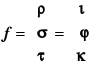The rotation is given by the expression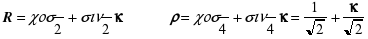The inverse of the quaternion is given by the expression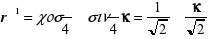The rest is simply algebra, being careful to keep the order of the products.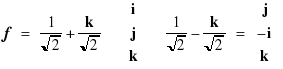This is precisely, what we reasoned from visualizing the rotation. So far, it has not been worth the effort of using quaternion analysis, but if we make the question a little more complex, then it becomes apparent that a formal structure is needed to express the rotation.